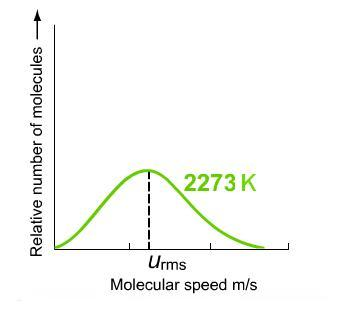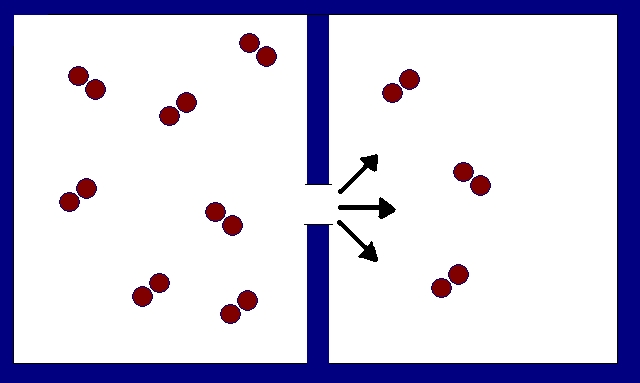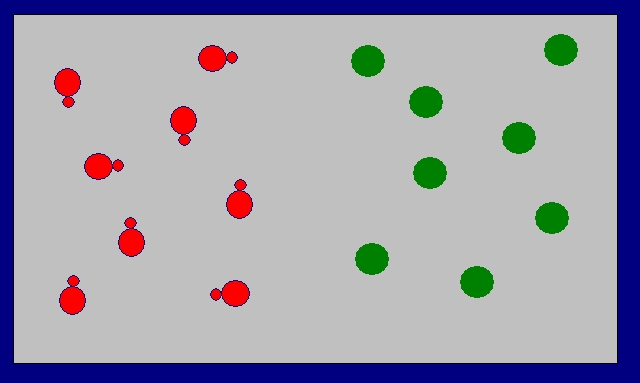# 6.6: How to Draw Enantiomers

$$\newcommand{\vecs}{\overset { \rightharpoonup} {\mathbf{#1}} }$$ $$\newcommand{\vecd}{\overset{-\!-\!\rightharpoonup}{\vphantom{a}\smash {#1}}}$$$$\newcommand{\id}{\mathrm{id}}$$ $$\newcommand{\Span}{\mathrm{span}}$$ $$\newcommand{\kernel}{\mathrm{null}\,}$$ $$\newcommand{\range}{\mathrm{range}\,}$$ $$\newcommand{\RealPart}{\mathrm{Re}}$$ $$\newcommand{\ImaginaryPart}{\mathrm{Im}}$$ $$\newcommand{\Argument}{\mathrm{Arg}}$$ $$\newcommand{\norm}{\| #1 \|}$$ $$\newcommand{\inner}{\langle #1, #2 \rangle}$$ $$\newcommand{\Span}{\mathrm{span}}$$ $$\newcommand{\id}{\mathrm{id}}$$ $$\newcommand{\Span}{\mathrm{span}}$$ $$\newcommand{\kernel}{\mathrm{null}\,}$$ $$\newcommand{\range}{\mathrm{range}\,}$$ $$\newcommand{\RealPart}{\mathrm{Re}}$$ $$\newcommand{\ImaginaryPart}{\mathrm{Im}}$$ $$\newcommand{\Argument}{\mathrm{Arg}}$$ $$\newcommand{\norm}{\| #1 \|}$$ $$\newcommand{\inner}{\langle #1, #2 \rangle}$$ $$\newcommand{\Span}{\mathrm{span}}$$$$\newcommand{\AA}{\unicode[.8,0]{x212B}}$$

When examining the ideal gas laws in conjunction with the kinetic theory of gases, we gain insights into the behavior of ideal gas. We can then predict how gas particles behaviors such as gas molecular speed, effusion rates, distances traveled by gas molecules. Graham's Law, which was formulated by the Scottish physical chemist Thomas Graham, is an important law that connects gas properties to the kinetic theory of gases.

## Introduction

The Kinetic Molecular Theory states that the average energy of molecules is proportional to absolute temperature as illustrated by the following equation:

$e_K=\dfrac{3}{2}\dfrac{R}{N_A}T$

where

• ek is the average translation kinetic energy,
• R is the gas constant,
• NA is Avogadro's number, and
• T is temperature in Kelvins.

Since R and NA are constants, this means that the Kelvin temperature (T) of a gas is directly proportional to the average kinetic energy of its molecules. This means that at a given temperature, different gases (for example He or O2) will the same average kinetic energy.

## Graham's Law

Gas molecules move constantly and randomly throughout the volume of the container they occupy. When examining the gas molecules individually, we see that not all of the molecules of a particular gas at a given temperature move at exactly the same speed. This means that each molecule of a gas have slightly different kinetic energy. To calculate the average kinetic energy (eK) of a sample of a gas, we use an average speed of the gas, called the root mean square speed (urms).

$e_K=\dfrac{1}{2}m{u_{rms}^2}$

with

• eK is the kinetic energy measures in Joules
• m is mass of a molecule of gas (kg)
• urms is the root mean square speed (m/s)

The root mean square speed, urms, can be determined from the temperature and molar mass of a gas.

$u_{rms}=\sqrt{\dfrac{3RT}{M}}$

with

• R the ideal gas constant (8.314 kg*m2/s2*mol*K)
• T temperature (Kelvin)
• M molar mass (kg/mol)

When examining the root mean square speed equation, we can see that the changes in temperature (T) and molar mass (M) affect the speed of the gas molecules. The speed of the molecules in a gas is proportional to the temperature and is inversely proportional to molar mass of the gas. In other words, as the temperature of a sample of gas is increased, the molecules speed up and the root mean square molecular speed increases as a result.Figure $$\PageIndex{1}$$: As the temperature of a gas sample increases from left to right, the molecular speed distribution increases indicate by the shift of the peaks form left to right from each graph.

Graham's Law states that the rate of effusion of two different gases at the same conditions are inversely proportional to the square roots of their molar masses as given by the following equation:

$\dfrac {\it{Rate\;of\;effusion\; of \;A} }{ \it{Rate \;of \;effusion\; of \;B}}=\dfrac{(u_rms)_A}{(u_rms)_B}=\dfrac{\sqrt {3RT/M_A}}{\sqrt{3RT/M_B}}=\dfrac{\sqrt{M_B}}{\sqrt{M_A}}$

In according with the Kinetic Molecular Theory, each gas molecule moves independently. However, the net rate at which gas molecules move depend on their average speed. By examining the equation above, we can conclude that the heavier the molar mass of the gas molecules slower the gas molecules move. And conversely, lighter the molar mass of the gas molecules the faster the gas molecules move.

##### Limitations of Graham's Law

Graham's Law can only be applied to gases at low pressures so that gas molecules escape through the tiny pinhole slowly. In addition, the pinhole must be tiny so that no collisions occur as the gas molecules pass through. Since Graham's Law is an extension of the Ideal Gas Law, gases that follows Graham's Law also follows the Ideal Gas Law.

## Molecular Effusion

The random and rapid motion of tiny gas molecules results in effusion. Effusion is the escape of gas molecules through a tiny hole or pinhole.Figure $$\PageIndex{1}$$: Illustration of gas molecules escaping through a small opening. This is a phenomenon called effusion.

The behavior of helium gas in balloons is an example of effusion. The balloons are made of latex which is porous material that the small helium atom can effuse through. The helium inside a newly inflated balloon will eventually effuse out. This is the reason why balloons will deflate after a period of time. Molecular speeds are also used to explain why small molecules (such as He) diffuse more rapidly than larger molecules (O2). That is the reason why a balloon filled with helium gas will deflate faster than a balloon filled with oxygen gas.Figure $$\PageIndex{1}$$: Example of effusion. The escaping of helium molecules from an inflated balloon causes the deflation of the balloon after a period of time.

The effusion rate, r, is inversely proportional to the square root of its molar mass, M.

$r\propto\sqrt{\dfrac{1}{M}}$

When there are two different gases the equation for effusion becomes

$\dfrac {\it{Rate\;of\;effusion\; of \;A} }{ \it{Rate \;of \;effusion\; of \;B}}=\dfrac{\sqrt {3RT/M_A}}{\sqrt{3RT/M_B}}$

$$M_A$$ is the molar mass of gas A, $$M_B$$ is the molar mass of gas B, $$T$$ Temperature in Kelvin, $$R$$ is the ideal gas constant.

From the equation above,

## Rates of effusion of two gases

The relative rates of effusion of two gases at the same temperature is given as:

$\dfrac{\it{Rate\;of\;effusion\;of\;gas\;}\mathrm 1}{\it{Rate\;of \;effusion\;of\;gas\;}\mathrm 2}=\dfrac{\sqrt{M_2}}{\sqrt{M_1}}$

Units used to express rate of effusion includes: moles/seconds, moles/minutes, grams/seconds, grams/minutes.

## Relative distances of two gases

The relative distances traveled by the two gases is given as:

$\dfrac{Distance\;traveled\;by\;gas\;1}{Distance\;traveled\;by\;gas\;2}=\dfrac{\sqrt{M_2}}{\sqrt{M_1}}$

By examine the Graham's law as stated above, we can conclude that a lighter gas will effuse or travel more rapidly than a heavier gas. Mathematically speaking, a gas with smaller molar mass will effuse faster than a gas with larger molar mass under the same condition.

## Molecular Diffusion

Similar to effusion, the process of diffusion is the spread of gas molecules through space or through a second substance such as the atmosphere.Figure $$\PageIndex{4}$$. The passage of one gas through another substance. In this example, the other substance is another gas.

Diffusion has many useful applications. Here is an example of diffusion that is use in everyday households. Natural gas is odorless and used commercially daily. An undetected leakage can be very dangerous as it is highly flammable and can cause an explosion when it comes in contact with an ignition source. In addition, the long term breathing of natural gas can lead to asphyxiation. Fortunately, chemists have discovered a way to easily detect natural gas occur leak by adding a small quantity of a gaseous organic sulfur compound named methyl mercaptan, CH3SH, to the natural gas. When a leak happens, the diffusion of the odorous methyl mercaptan in the natural gas will serve as a sign of warning.

## General guideline in solving effusion problems

The following flowchart outlines the steps in solving quantitative problems involving Graham's Law. It can be used as a general guideline.##### Example $$\PageIndex{1}$$

Calculate the root mean square speed, $$u_{rms}$$, in m/s of helium at $$30 ^o C$$.

###### Solution

Start by converting the molar mass for helium from g/mol to kg/mol.

$$M=(4.00\;g/mol)\times\dfrac{1\;kg}{1000\;g}$$

$$M=4.00\times10^{-3}\;kg/mol$$

Now, using the equation for $$u_{rms}$$ substitute in the proper values for each variable and perform the calculation.

$u_{rms}=\sqrt{\dfrac{3RT}{M}}$

$u_{rms}=\sqrt{\dfrac{3 \times (8.314\; kg\;m^2/s^2*mol*K)(303K)}{4.00 \times 10^{-3}kg/mol}}$

$u_{rms}=1.37 \times 10^3 \;m/s$

##### Example $$\PageIndex{2}$$

What is the ratio of $$u_{rms}$$ values for helium vs. xenon at $$30^oC$$. Which is higher and why?

###### Solution

There are two approaches to solve this problem: the hard way and the easy way

Hard way:

The $$u_{rms}$$ speed of helium is calculated from the above example.

First convert the molar mass of xenon from g/mol to kg/mol as we did for helium in example 1

$$M_{Xe}=(131.3\;g/mol)\times\dfrac{1\;kg}{1000\;g}$$

$$M=0.1313\;kg/mol$$

Now, using the equation for the urms, insert the given and known values and solve for the variable of interest.

$u_{rms}=\sqrt{\dfrac{3(8.314\; kg\;m^2/s^2*mol*K)(303\;K)}{0.1313\;kg/mol}}$

$u_{rms}=2.40 \times 10^2\;m/s$

Compare the two values for xenon and helium and decide which is greater.

• $$u_{Xe}=2.4 \times 10^2 \;m/s$$
• $$u_{He}=1.37 \times10^3 \;m/s$$

So the ratio of RMS speeds is

$\dfrac{u_{Xe}}{u_{He}} \approx 0.18$

Helium has the higher $$u_{rms}$$ speed. This is in according with Graham's Law, because helium atoms are much lighter than xenon atoms.

Easy Way:

Since the temperature is the same for both gases, only the square root of the ratio of molar mass is needed to be calculated.

$\sqrt{\dfrac{M_{He}}{M_{Xe}}} = \sqrt{\dfrac{4.00 \; g/mol}{131.3\;g/mol }} \approx 0.18$

In either approach, helium has a faster RMS speed than xenon and this is due exclusively to its smaller mass.

##### Example $$\PageIndex{3}$$

If oxygen effuses from a container in 5.00 minutes, what is the molecular weight of a gas with the same given quantity of molecules effusing from the same container in 4.00 min?

###### Solution

Let oxygen be gas A and its rate is 1/5.00 minutes because it takes that much time for a certain quantity of oxygen to effuse and its molecular weight is 32 grams/mole (O2 (g)). Let the unknown gas be B and its rate is 1/4.00 minutes and Mb be the molecular weight of the unknown gas.

First, choose the appropriate equation,

$$\dfrac{\it{Rate\;effusion\;of\;gas\;A}}{\it{Rate\;effusion\;of\;gas\;B}}=\dfrac{\sqrt{M_B}}{\sqrt{M_A}}$$

Second, using algebra, solve for the variable of interest on one side of the equation and plug in known and given values from the problem.

$$\sqrt{M_B}=\dfrac{(\mathit{Rate\;effusion\;of\;gas\;A} )(\sqrt{M_A})}{\mathit{Rate\;effusion\;of\;gas\;B}}$$

$$\sqrt{M_B}=\dfrac{(1/5.00\;minutes)(\sqrt{32\;grams/mole})}{1/4.00\;minutes}$$

$$\sqrt{M_B}=4.525$$

$${M_B}=(4.525)^2=20.5\;grams/mole$$

##### Example $$\PageIndex{4}$$

Oxygen, O2 (g), effuses from a container at the rate of 3.64 mL/sec, what is the molecular weight of a gas effusing from the same container under identical conditions at 4.48 mL/sec?

###### Solution

Let label oxygen as gas A. Therefore, rate of effusion of gas A is equaled to 3.64 mL/sec and the molecular weight is 32 grams/mole. The unknown gas is B and its rate of effusion is 4.48 mL/sec. We are solving for the molecular weight of gas B which is labeled as MB.

First, choose the appropriate equation,

$$\dfrac{\it{Rate\;effusion\;of\;gas\;A}}{\it{Rate\;effusion\;of\;gas\;B}}=\dfrac{\sqrt{M_B}}{\sqrt{M_A}}$$

$$\sqrt{M_B}=\dfrac{(\mathit{Rate\;effusion\;of\;gas\;A} )(\sqrt{M_A})}{\mathit{Rate\;effusion\;of\;gas\;B}}$$

Second, using algebra, solve for the variable of interest on one side of the equation and plug in known and given values from the problem.

$$\sqrt{M_B}=\dfrac{(\mathit{Rate\;effusion\;of\;gas\;A})(\sqrt{M_A})}{\mathit{Rate\;effusion\;of\;gas\;B}}$$

$$\sqrt{M_B}=\dfrac{(3.64\;mL/sec)(\sqrt{32\;grams/mole})}{4.48\;mL/sec}$$

$$\sqrt{M_B}=4.50$$

$${M_B}=(4.50)^2=20.2\;grams/mole$$

##### Example $$\PageIndex{5}$$

Which answer(s) are true when comparing 1.0 mol O2 (g) at STP (Standard Temperature and Pressure) and 0.50 mole of S2 (g) at STP?

The two gases have equal through the same orifice, a tiny opening:

1. average molecular kinetic energies
2. root-mean-square speeds
3. masses
4. volumes
5. densities
6. effusion rates
###### Solution

The average kinetic energy of gas molecules depends on only the Temperature as exemplifies in this equation:

$$e_K=\dfrac{3}{2}\dfrac{R}{N_A}T$$

In addition, the mass of 0.50 mole of S2 is the same as that of 1.0 mole of O2. The rest of the choices are false.

Therefore, the correct answers are (a) and (c).

## References

1. Petrucci, RH et al. (2007). General Chemistry: Principles and Modern Applications. New Jersey: Pearson Prentice Hall. p. 201-209.
2. Oxtoby, DW et al. (2008). Principles of Modern Chemistry. Belmont, California: Thomson Brooks/Cole. p.379-386
3. Gilbert, TR et al. (June 2003). Chemistry: The Science in Context. New York: W.W. Norton & Company. Chapter 8

6.6: How to Draw Enantiomers is shared under a not declared license and was authored, remixed, and/or curated by LibreTexts.